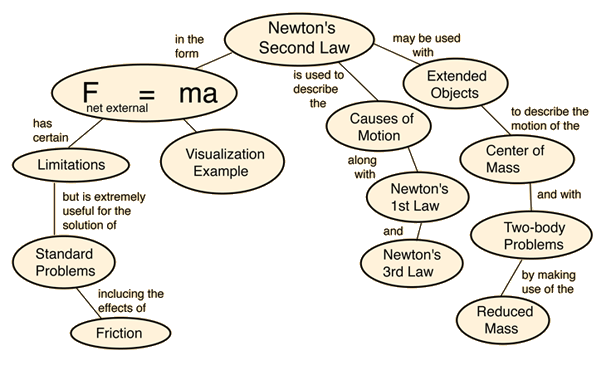## Newton's three laws of motion (simplified) are:

1. An object in motion/rest stays in motion/rest.
2. The direction of the force is the same as the direction of acceleration.
3. Every action has an equal and opposite reaction.

## More in-depth look at each law of motion

1. Every object persists in its state of rest or uniform motion in a straight line unless it is compelled to change that state by forces impressed on it.

Example:

• A stationary ball will stay in the same position unless there is a fore put onto the ball.

2. Force is equal to the change in momentum (mV) per change in time. For a constant mass, force equals mass times acceleration. In this law the direction of the force vector is the same as the direction of the acceleration vector.

The relationship between an object's mass, its acceleration, and the applied force.

F= m * a

Example:

• If you push a stationary ball with a force greater then the mass of the ball, the ball will move in the direction the force was applied.

3. For every action, there is an equal and opposite re-action.

Example:

• If a stationary ball is hit by another ball in motion, the motion is tranferred from the ball in motion to the stationary ball. The stationary ball will move in the opposite direction from which the force come from with the equal amount of movement.

## Relevency:

The laws of motion take place in all situation on all objects.

The Saskachewan Curriculum requiers the intorduction to motion in grade two's physical science providing the foundation for further development build onto it.

Outcomes:

 MP2.2 Investigate factors, including friction, which affect the motion of natural and constructed objects, including self. (SI)
 FM5.1 Analyze the effects of gravitational, magnetic, and mechanical forces, including friction, on the movement of objects. (CP, SI)

The mind map below demonstaights interactions and applications of the three laws.http://hyperphysics.phy-astr.gsu.edu/hbase/Newt.htmlhttp://hyperphysics.phy-astr.gsu.edu/hbase/Newt.html

Use the links above to visit the interactive version of the images.

References

Hall, N. (2015, May 5). Newton's Laws of Motion. Retrieved from Glenn Research Center & National Aeronautics and Space Administration: https://www.grc.nasa.gov/www/k-12/airplane/newton.html

Nave, C. R. (2016, August). HyperPhysics: Mechanics. Retrieved from Georgia State University: Department of Physics and Astronomy: http://hyperphysics.phy-astr.gsu.edu/hbase/hframe.html